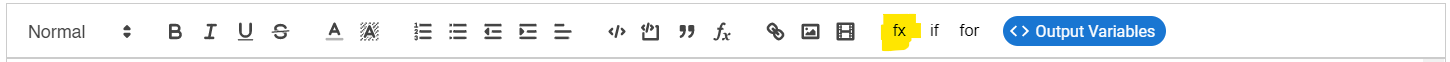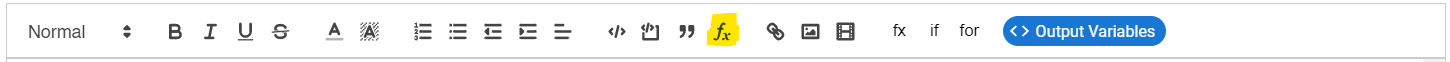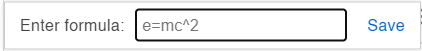# Latex

MecSimCalc uses KateX for displaying latex equations within the Rich Text and Output in-browser editors.

## Using Katex filter​

| katex is a special filter that converts the string to its left into Katex/Latex when the app is executed.

### String​

A latex formula written in a string can be inserted using the following form:

{{ "latex expression" | katex }}
caution

Unlike traditional latex expressions, the character \ should be replaced with \\. For example, the following expression is used to display the equation that follows:

$P=\frac{2 \sigma_y t}{OD} \times F \times L \times J \times T$

{{ "P=\\frac{2 \\sigma_y t}{OD} \\times F \\times L \\times J \\times T" | katex }}

### Python Variable​

Alternatively, the developer can choose to return a latex expression as a string from the main function in the Code step.

When using the \ character in the Python string, you need to either:

• Escape the character by using \\ instead of \.
• Or use a raw string, which allows you to only use \.
• A raw string is simply created by adding the character r before the string quotation marks. For example: r"this is a raw string"

Once the latex string is returned from the main function, it must be embedded into the Output step using the | katex filter. For example:

{{ outputs.math_equation | katex }}
tip

Click the fx button in the toolbar to quickly insert {{ "x^2" | katex }}### Example​

As a demonstration, this app provides an example where the user inputs a value for $y$, and the app then displays the following equation using three different methods: $y=value=\frac{x^2+f(x)+\log(x)}{\cos(x)}$

The Python code for this app has the form:

def main(inputs):    y = inputs['y']    string1 = "y=" + str(y) + "=\\frac{x^2+f(x)+\\log(x)}{\\cos{x}}"    string2 = "y=" + str(y) + r"=\frac{x^2+f(x)+\log(x)}{\cos{x}}"    return {"Regular String": string1, "Raw String": string2, "y":y}

The following is the output editor text:

Equation output using regular string:{{ outputs["Regular String"] | katex }}Equation output using raw string:{{ outputs["Raw String"] | katex }}Equation output using output editor:{{ "y=" | katex }}{{ outputs.y | katex }}{{ "=\\frac{x^2+f(x)+\\log(x)}{\\cos(x)}" | katex }}

## Equation button​

Another way to insert a latex equation that does not change is to click on the $f_x$ button in the toolbar:Then, a popup will appear where you can write your latex expression. In this case, you can simply use \ instead of \\.Once your equation is done, click Save and a rendered version of the equation will appear.

danger

Once the equation is saved, it can not be edited. Therefore, if your equation is very complex, then it is strongly recommended to either write the latex expression down somewhere or to use the | katex filter described above.